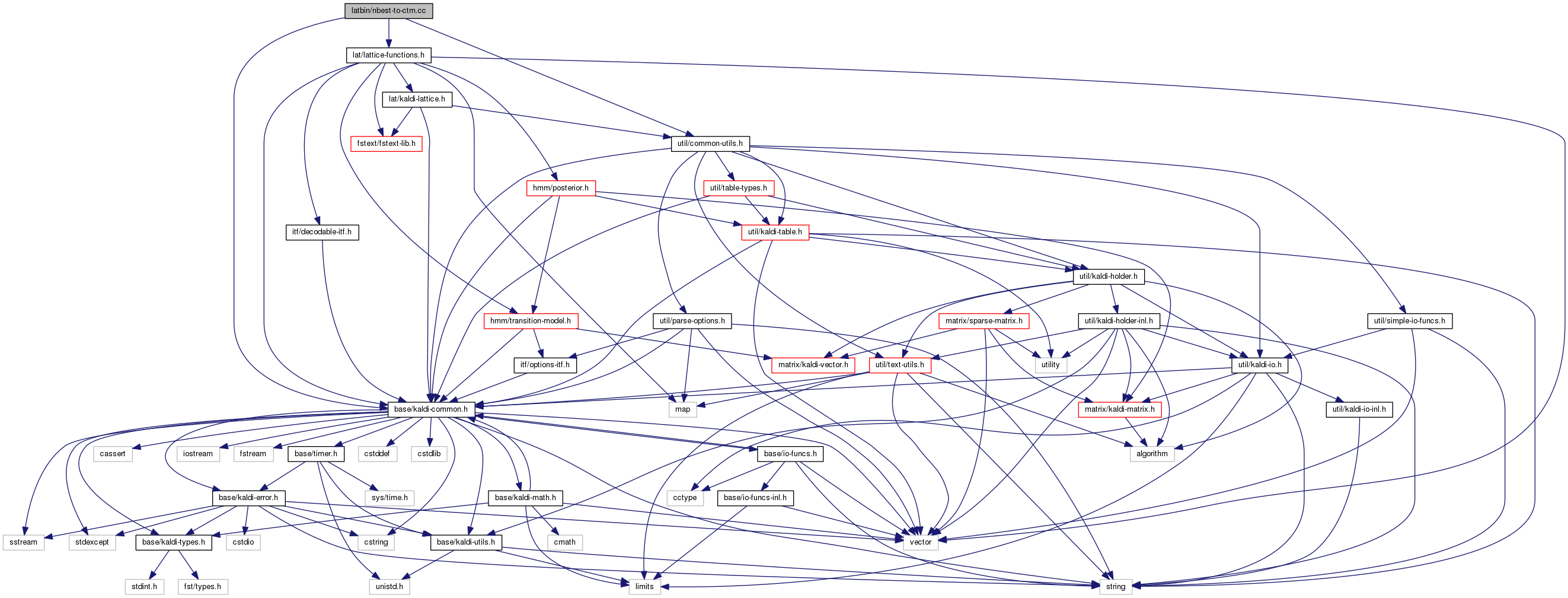nbest-to-ctm.cc File Reference
`#include "base/kaldi-common.h"`
`#include "util/common-utils.h"`
`#include "lat/lattice-functions.h"`
Include dependency graph for nbest-to-ctm.cc:Go to the source code of this file.

## Functions

int main (int argc, char *argv[])

## ◆ main()

 int main ( int argc, char * argv[] )

Definition at line 25 of file nbest-to-ctm.cc.

25  {
26  try {
27  using namespace kaldi;
28  typedef kaldi::int32 int32;
29
30  const char *usage =
31  "Takes as input lattices which must be linear (single path),\n"
32  "and must be in CompactLattice form where the transition-ids on the arcs\n"
33  "have been aligned with the word boundaries... typically the input will\n"
34  "be a lattice that has been piped through lattice-1best and then\n"
35  "lattice-align-words. On the other hand, whenever we directly pipe\n"
36  "the output of lattice-align-words-lexicon into nbest-to-ctm,\n"
37  "we need to put the command `lattice-1best ark:- ark:-` between them,\n"
38  "because even for linear lattices, lattice-align-words-lexicon can\n"
39  "in certain cases produce non-linear outputs (due to disambiguity\n"
40  "in the lexicon). It outputs ctm format (with integers in place of words),\n"
41  "assuming the frame length is 0.01 seconds by default (change this with the\n"
42  "--frame-length option). Note: the output is in the form\n"
43  "<utterance-id> 1 <begin-time> <end-time> <word-id>\n"
44  "and you can post-process this to account for segmentation issues and to \n"
45  "convert ints to words; note, the times are relative to start of the utterance.\n"
46  "\n"
47  "Usage: nbest-to-ctm [options] <aligned-linear-lattice-rspecifier> <ctm-wxfilename>\n"
48  "e.g.: lattice-1best --acoustic-weight=0.08333 ark:1.lats | \\\n"
49  " lattice-align-words data/lang/phones/word_boundary.int exp/dir/final.mdl ark:- ark:- | \\\n"
50  " nbest-to-ctm ark:- 1.ctm\n"
51  "e.g.: lattice-align-words-lexicon data/lang/phones/align_lexicon.int exp/dir/final.mdl ark:1.lats ark:- | \\\n"
52  " lattice-1best ark:- ark:- | \\\n"
53  " nbest-to-ctm ark:- 1.ctm\n";
54
55  ParseOptions po(usage);
56
57  bool print_silence = false;
58  BaseFloat frame_shift = 0.01;
59  int32 precision = 2;
60  po.Register("print-silence", &print_silence, "If true, print optional-silence "
61  "(<eps>) arcs");
62  po.Register("frame-shift", &frame_shift, "Time in seconds between frames.\n");
63  po.Register("precision", &precision,
64  "Number of decimal places for start duration times (note: we "
65  "may use a higher value than this if it's obvious from "
66  "--frame-shift that this value is too small");
67
69
70  if (po.NumArgs() != 2) {
71  po.PrintUsage();
72  exit(1);
73  }
74
75  std::string lats_rspecifier = po.GetArg(1),
76  ctm_wxfilename = po.GetArg(2);
77
78  if (frame_shift < 0.01 && precision <= 2)
79  precision = 3;
80  if (frame_shift < 0.001 && precision <= 3)
81  precision = 4;
82
83
85
86  int32 n_done = 0, n_err = 0;
87
88  Output ko(ctm_wxfilename, false); // false == non-binary write mode.
89  ko.Stream() << std::fixed; // Set to "fixed" floating point model, where precision() specifies
90  // the #digits after the decimal point.
91  ko.Stream().precision(precision);
92
96
97  std::vector<int32> words, times, lengths;
98
99  if (!CompactLatticeToWordAlignment(clat, &words, &times, &lengths)) {
100  n_err++;
101  KALDI_WARN << "Format conversion failed for key " << key;
102  } else {
103  KALDI_ASSERT(words.size() == times.size() &&
104  words.size() == lengths.size());
105  for (size_t i = 0; i < words.size(); i++) {
106  if (words[i] == 0 && !print_silence) // Don't output anything for <eps> links, which
107  continue; // correspond to silence....
108  ko.Stream() << key << " 1 " << (frame_shift * times[i]) << ' '
109  << (frame_shift * lengths[i]) << ' ' << words[i] <<std::endl;
110  }
111  n_done++;
112  }
113  }
114  ko.Close(); // Note: we don't normally call Close() on these things,
115  // we just let them go out of scope and it happens automatically.
116  // We do it this time in order to avoid wrongly printing out a success message
117  // if the stream was going to fail to close
118
119  KALDI_LOG << "Converted " << n_done << " linear lattices to ctm format; "
120  << n_err << " had errors.";
121  return (n_done != 0 ? 0 : 1);
122  } catch(const std::exception &e) {
123  std::cerr << e.what();
124  return -1;
125  }
126 }
int32 words[kMaxOrder]
This code computes Goodness of Pronunciation (GOP) and extracts phone-level pronunciation feature for...
Definition: chain.dox:20
kaldi::int32 int32
float BaseFloat
Definition: kaldi-types.h:29
The class ParseOptions is for parsing command-line options; see Parsing command-line options for more...
Definition: parse-options.h:36
A templated class for reading objects sequentially from an archive or script file; see The Table conc...
Definition: kaldi-table.h:287
#define KALDI_WARN
Definition: kaldi-error.h:150
fst::VectorFst< CompactLatticeArc > CompactLattice
Definition: kaldi-lattice.h:46
#define KALDI_ASSERT(cond)
Definition: kaldi-error.h:185
#define KALDI_LOG
Definition: kaldi-error.h:153
bool CompactLatticeToWordAlignment(const CompactLattice &clat, std::vector< int32 > *words, std::vector< int32 > *begin_times, std::vector< int32 > *lengths)
This function takes a CompactLattice that should only contain a single linear sequence (e...